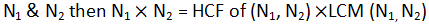# Ratio and Proportion Questions & Answers### Question. 1.

In a mixture of 40 litres, the ratio of milk and water is 4:1. How much water must be added to this mixture so that the ratio of milk and water becomes 2:3.
(a) 20 litres
(b) 32 litres
(c) 40 litres
(d) 30 litres

### Question. 2.

If three numbers are in the ratio of 1:2:3 and half the sum is 18, then the ratio of squares of the numbers is:
(a) 6: 12: 13
(b) 1: 2: 4
(c) 36: 144: 324
(d) None of these

### Question. 3.

The ratio between the two numbers is 3:4 and their LCM is 180. The first number is:
(a) 60
(b) 45
(c) 15
(d) 20

### Question. 4.

The incomes of A and B are in the ratio 3:2 and their expenditures are in the ratio 5:3. If each saves Rs. 1000, then, A's income is
(a) Rs. 3000
(b) Rs. 4000
(c) Rs. 6000
(d) Rs. 9000

### Question. 5.

The students in three batches at Made Easy are in the ratio 2 : 3 : 5. If 20 students are increased in each batch, the ratio change to 4:5:7. The total number of students in the three batches before the increase were.
(a) 10
(b) 90
(c) 100
(d) 150

### Question. 6.

The difference between the two positive numbers is 10 and the ratio between them is 5:3. Find the product the two numbers
(a) 375
(b) 175
(c) 275
(d) 125

### Question. 7.

Four numbers in the ratio 1:3:4:7 add up to give a sum of 105. Find the value of the biggest number
(a) 42
(b) 35
(c) 49
(d) 63

### Question. 8.

If Rs. 58 is divided among 150 children such that each girl and each boy gets 25 p and 50 p respectively. Then how many girls are there?
(a) 52
(b) 54
(c) 68
(d) 62

## Solutions:

#### Ans 1. (c)

SolutionsLet water is x
4 x : x = milk : water
32: 8 = 4x : x (since total 40 liters)#### Ans 2.(c)

Let numbers are
x : 2x : 3x also it is given that
6x = 36, x = 6
6 : 12 : 18
The ratio of squares 36: 144 : 324: or also 1 : 4 : 9

#### Ans 3. (b)

Let number are 3x and 4x
3x 4x =HCF ✕ 180
Clearly, HCF will be x
12x² = x 180, x = 15
Number will be 45:60
Note: if there are two number#### Ans 4.(c)

Let incomes are 3x:2x
Expenditures are 5y:3y
Then
3x­­–5y = 1000            …..(i) also
2x–3y = 1000                   …..(ii)
from (i) and (ii) we get
6x-10y = 2000
+6x-9y = 3000
-y =-1000
y=1000, x= 2000
A's income is 6000

#### Ans 5.(c)

let students are 2x: 3r: 5x now According to the given condition
2x+20 : 3x+20 : 5x + 20 = 4 : 5 : 7
x = 10
20:30:50 = 2:3:5 and after adding 20
40:50:70

#### Ans 6. (a)

x-y = 10              ……(i)
x:y = 5:3            ……(ii)
x:y = 5t:3t         ……(iii)
according to (i) and (iii)
5t-3t = 10
2t = 10, t = 5
x = 25, y = 15
Product of two numbers = 375

#### Ans 7.(c)

x : 3x : 4x : 7x
re four numbers also
x + 3x + 4x + 7x = 105
15x = 105, x = 7, 7r = 49

#### Ans 8.(c)

Let the number of girls and boys are x and y then
0.25x + 0.5 = 58         ….(i)
also
x + y = 150                 ….(ii)
from (i) and (ii) we get
0.5x + y = 116
x + y = 150
So, 0.5x = 34, x = 68, y = 82